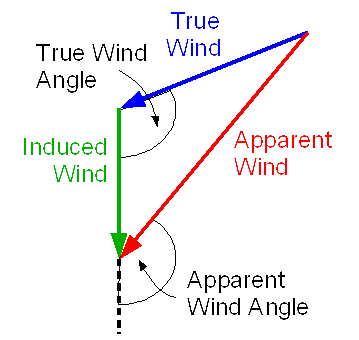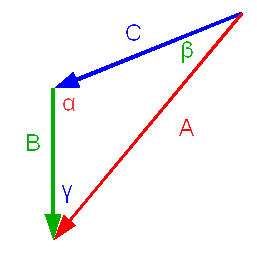# Sailing Maths

Here is an explanation of some of the maths behind sailing.

Looking at the diagram for VMG we can see that this is a right angled triangle, so we can solve this with some simple trigonometry.We know:

• Boat Speed - this is the hypotenuse
• Course Offset Angle

We want to find:

• VMG – this is the side adjacent to the Course Offset Angle.

For a right angled triangle:

So

This gives us the formula for VMG for a given boat speed and course offset:

VMG = cos(Course Offset Angle).(Boat Speed).

## Apparent Wind

If you read any primer on sailing you’ll find a diagram like this explaining apparent wind.In general this is not a right angled triangle so we have to use the cosine rule to solve it (in some cases the sine rule is used for this sort of triangle, but for the information that we know the cosine rule is the one to use).We define a triangle to have sides of lengths A, B and C. In this triangle the we call the angle opposite side A α, the angle opposite side B β and the angle opposite side C γ. The cosine rule states that:

A 2 = B 2 + C 2 – 2.B.C.Cos(α)

B 2 = A 2 + C 2 – 2.A.C.Cos(β)

C 2 = A 2 + B 2 – 2.A.B.Cos(γ)

For a given boat speed, true wind strength and true wind angle (relative to the boat’s head) we want to find what the apparent wind speed is and the angle of the apparent wind (again relative to the boat’s head).

We know:

• Boat Speed – call this “B”
• True Wind Speed – call this “C”
• True Wind Angle

The true wind angle is measured from the boat’s head, we actually want the angle inside the triangle between sides B and C (angle α), which is 180º - True Wind Angle.

Lets start by finding:

• Apparent Wind Speed – which is side A

We can apply the cosine rule directly:

A 2 = B 2 + C 2 – 2.B.C.Cos(α)

So A = SQR(B 2 + C 2 – 2.B.C.Cos(α))

In other words:

Apparent Wind Speed = SQR((Boat Speed) 2 + (True Wind Speed) 2 – 2.(Boat Speed).(True Wind Speed).Cos(180º - True Wind Angle)

Now we know all three sides of the triangle we can work out the apparent wind angle, which is angle γ

C 2 = A 2 + B 2 – 2.A.B.Cos(γ)

So:

γ = Cos -1 ((A2 + B2 – C2)/(2.A.B))

In other words:

Apparent Wind Angle = Cos -1 ((Apparent Wind Speed) 2 + (Boat Speed) 2 – (True Wind Speed) 2 )/(2.(Apparent Wind Speed).(Boat Speed)))

## True Wind

When we’re sailing we can measure the apparent wind and the boat speed and use them to calculate the true wind (this is the maths that integrated instruments use to display true wind).

We know:

• Apparent Wind Speed – call this “A”
• Apparent Wind Angle – call this “β”
• Boat Speed – call this “B”

Lets start by finding:

• True Wind Speed – which is side “C”

We can apply the cosine rule directly:

C 2 = A 2 + B 2 – 2.A.B.Cos(γ)

So:

C = SQR(A 2 + B 2 – 2.A.B.Cos(γ))

In other words:

True Wind Speed = SQR((Apparent Wind Speed) 2 + (Boat Speed) 2 – 2.(Apparent Wind Speed).(Boat Speed).Cos(Apparent Wind Angle))

Next we find the True Wind Angle, which is (180º - α):

A 2 = B 2 + C 2 – 2.B.C.Cos(α)

So:

α = Cos -1 ((B 2 + C 2 – A 2 )/( 2.B.C))

True Wind Angle = 180º - α

In other words:

True Wind Angle = 180º - Cos -1 (((Boat Speed) 2 + (True Wind Speed) 2 – (Apparent Wind Speed) 2 )/( 2.(Boat Speed).(True Wind Speed)))